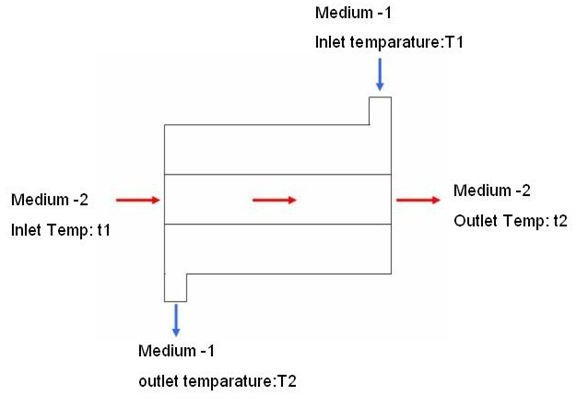# What is an Air to Air Heat Recovery Exchanger: Heat Exchanger Design Basics

## What is a Heat Exchanger?

A heat exchanger is equipment used for transferring heat from one medium to another. The picture below schematically represents the construction of a simple heat exchanger:In a heat exchanger, heat gets transferred from a hot fluid (Medium-2) to a cold fluid (Medium-1).

## What is a Air to Air Heat Exchanger?

If the fluid pass through the heat exchanger is air, then the heat exchanger is called an air to air heat exchanger. Both Medium-1 as well as Medium-2 are air in case of air to air heat exchanger. Hot air passes through the tubes and while doing so, it exchanges heat with the flow of cold air.

## Guidelines for Air to Air Heat Exchanger Design

Following are the typical steps for an air to air heat exchanger design (Please refer the above attached picture):

• Select heat exchanger type (i.e. decide number of passages).
• Find out the inlet and outlet temperatures (t1, t2, T1 and T2) of both the sides of the heat exchanger. These values are specified based on the application of the air to air heat exchanger.
• Calculate heat load by using the following formulae:

H = m1 * c1 * (T2 – T1) = m2 * c2 * (t1 – t2) ……..Eqn.1

Where,

H – Total heat load of the heat exchanger.

m1 & m2 – Mass of air (flowing per unit time) for side 1 & 2 respectively.

c1 & c2 – Specific heat of air flowing in side 1 & 2 respectively.

• Calculate LMTD (Log Mean Temperature Difference) by using the following formula:

LMTD = [ (T1 – t2) – (t1 – T2) ]/[ ln (T1 – t2) /(t1 – T2) ]…….Eqn.2

• Find out the value of overall heat transfer coefficient (U) using the following formula:

1/U = 1/h1 + w/K + 1/h2………..Eqn.3

Where,

U – Overall heat transfer coefficient of the air to air heat exchanger.

h1 & h2 – Convection heat transfer coefficient air of both the sides of the heat exchanger.

K – Thermal conductivity of the tube material.

• Apply the following equation to calculate required surface area:

H = U*A*LMTD……Eqn.3

Where,

A – Required surface area.

Once we got the total required surface area, we can size the air to air heat exchanger.

## Conclusion

Air to air heat exchanger is a special type of heat exchanger which carries air as primary as well as secondary medium. Basic procedures for heat exchanger design discussed here will be helpful for complex practical heat exchangers.